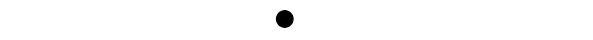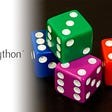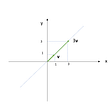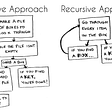# ABZeus

direction towards the start of everything from existing forces to existing elements

# 1 | The minimal representation

The minimal representative entity that could possible be depicted to transmit an idea, together with the concepts of duality and trinity, are the three main ideas bounded within the roots of this work.

# 1.1 | The minimal representative entityThere is no other known glyph to transmit the idea of something that can be accounted for. For the rest of this work, the dot, serves as the idea of an element, which in ABZeus translates as ‘ l men t ’; direction of a minimal representable entity from many to singulars which exists.

# 1.2 | The dualityAnalog to the dualism between matter and energy in Physics, In order to capture the dualism in the interpretation of our reality; the * glyph is used to transmit the opposite concept to the minimal representable entity, nevertheless, both together representing the idea of everything.

Rooted on the idea of quantifying the information around an observer in a bi dimensional plane; if we graph this hypothesis where the observer is in the center, represented by the dot, it will be surrounded by eight quadrants holding definable information towards all directions around the observer, although, losing its definition towards all directions until this information is insignificant or completely absent, therefore undefined. The asterisk represents the idea of the eight directions towards the observer.

# 1.3 | The trinityFor any minimal representative entity in one dimension there will be forward and backward, where the sum of all entities are always three.

Through language is possible to describe a minimum interpretable universe, in a model that could describe a Universe in any dimension in a subjective way from an information point of view.

Depicting a minimum interpretable information universe:

On a unidimensional container without specifying its edges nor limits we can describe only an empty container, and within this empty container a minimal one dimensional representable entity which has access to the information forward and backward to itself.

Through this diagram I want to express the idea that for any point of information there is something forward and backwards to the observer, and for a minimum viable universal interpretation of information any observer or minimal information representative entity will consider the undefined forward and previously to itself. Being three, the sum of all quantifiable entities in any minimal representable system.

If we extrapolate this idea into a bidimensional information universe, then we will say that the minimal information representative entity has access to all the information in two axis (x,y). And if we want to represent this information in a diagram, the unidimensional diagram contains only 3 quantifiable units of information, and in a bidimensional diagram will contain 9 quantifiable units of information, basically c to the power of 2.

Now we have a minimum viable interpretable information universe in a subjective way, considering that the minimum amount of quantifiable entities to interpret and describe any unidimensional system will always be c = 3.

At this moment I have expressed the idea that ‘something’ always has ‘something’ around in all directions, and only quantifying in terms of language the amount of elements to give a minimum interpretation which is c² in a bidimensional system.

# 2 | Num — Rationals from singulars to plurals

Rational (u) from singulars (n) to plural (m).

# 2.1 | One

## singular defined element

singular from the defined to the minimally representable

In its beginning the one is represented by a dot, which symbolises the minimal information representable entity, nevertheless, later is replaced by the vertical bar, which transmit the idea of a limit that express the singularisation of what can be defined and quantified. Symbolically, it represents something describable in its totality as a singular group of rationals from singulars to plurals as a whole, furthermore it also transmit the idea of the describable Self as the observer, if we situate the Self as the observer in the center of this interpretation.

# 2.2 | Two

Originally the number two logically represents the quantitation of the elements involved within the dualism fundaments of this work.

If we start with the concept of the the dot as the minimal representative entity, the logical pattern will be to depict two dots ( : ); Nevertheless, following the philosophical pattern in this work related to the dualism, the number should follow the ( .* ) pattern, which establishes the minimal representation of the duality will be the minimal representative entity towards the most holistic and abstract representation.

From the objective towards the subjective, both together as the whole.

Nevertheless, after conceiving all the quantifiable elements which forms the numbers, this work conclude that the best representation to describe ONE is I, because it represents the singularisation of the digits within the defined, therefore at this point the most logical would be to represent TWO as II following a successive addition pattern, being the generally adopted symbol to describe 2.

## The origins of 2

The word TWO, in ABZeus translates as (+Ω O ) : ‘Everything from what exists to the defined’. In a similar approach, the word could be depicted as
( . -O- * ): Everything from the minimal representable to the most abstract representation.

The approach used in this work, attempts to transmit the idea behind the dualism as everything, using -O-, or Ω to express the idea of absolutely everything, including the undefined. Finally to express the idea of TWO as the division of Ω, %Ω; 2.

Both parts complementing the everything in dualistic parts, the beginning and the end, the objective and the subjective, etc, expressed by the letters A, and B.

Before the number symbolical representation, this approach gives birth to the ABZeus B.

# 2.3 | Three

For any minimal representative entity in one dimension there will be forward and backward, where the sum of all entities are always three.

The ‘trinity’ philosophy gives its entire meaning to the number 3; it transmit the idea that for any representable entity there will be always two directions, in this case limits.

For this is necessary to understand the meaning of the two glyphs involved in the figure. Element and Limit.

# ☐ ■ ☐

In a minimum interpretable information system three entities can be defined, the minimal representable entity and what exists forward and previously to it.

[ q , I , p ] = ω = oo

• |, 1, I
( One ) Defined singular element
• ||, 2, II
Omega division. Ω|2, reference to ‘q’ or ‘p’
• |.|, 3, III
‘q’ + ‘I’ + ‘p’ = ω = oo

# 2.5 | IV, V, VI, VII , VIII — Two dimensional numbers

☒ ☐ ☒

☒ ■ ☒

☒ ☐ ☒

They represent everything that can be quantified in two dimensions, excluding the observer. Symbolically numbers 4, 5, 6 and 7 and 8 ( * ), are formed by additions and combinations of the symbol V which represents a ‘portion’.

# 2.6 | Eight

The number 8, represents the group of portions quantised around the minimal representable entity in a bi dimensional plane, which becomes representation of the undefined within information terms, or infinite towards all directions from the minimal representable entity point of view.

In its original form, the number 8 is represented by the asterisk (*), which is the representation of the eight directions surrounding a minimal representative entity in a bi dimensional plane.

It also represents the idea of everything around the minimal representative entity, nevertheless if it is in one dimension or two dimensions, therefore for one dimension the figure of 8, responds to oo, which is a + b.

☒ ☒ ☒

☒ ■ ☒

☒ ☒ ☒

# 2.7 | Nine

## Singularly describable minimal representable entity

Representing a singular rational defined within the defined, symbolising the presence of the observer within the defined, or only referring to the group of quantifiable entities within the defined, excluding its inherit association with the defined, as the content, not the group.

Also represents the Self within Everything. The number 9, could be a symbolic metaphor to the moment of an ovule fecundation. In Greek symbology, IX, also represent the Self within the 2 dimensional plane denoted by X, which also denotes position; a.k.a, the describable that is here.

# 2.8 | Zero

## Magnitud limit of the radio from the minimal representative entity towards the defined.

Representing a non quantifiable entity of rationals from singulars to plurals, which represents in its whole all that is defined.

# 2.8.1 | Third dimensional number

10 is a tridimensional number, which denotes the imaginary group of 9 entities which conforms the bi-dimensional plane of 9, including the observer.

O is a non quantifiable group, as it represents an imaginary string that groups measurable contents.

The form is described in the pictogram as the group of numerals from 1 to 9, except that 8 and 9 includes the form.

Numerals from 1 to 3 describes all the rational quantifiable units in a one dimensional plane.

Numerals from 4 to 8 describes all the rational quantifiable units in a bi dimensional plane around an element.

Number 8, represents all the rational quantifiable units around the element and is formed by the concept of a + b. This could be the reason, because 8 and 9 contains the convex group symbol O, where philosophically a represents the concept of the imaginary group that contains all the measurable content, connected to something else; so a = -o, and b = o-.

Pictographically by adding O- and -O, where O- = a, -O = b.

The number 9 represents a minimal representable entity surrounded by 8 directions in a bi dimensional plane.

Finally the number 0, is a non quantifiable unit, represented by the concept of a group, acting as an additive identity.

Zero = ( Z = magnitude limit ) + ( e (adj.), r (subject), (o (adj.) ). ( ero = radius convex elements ) . : magnitude limit of radius convex elements.

# 3 | Nom

Defined from singulars to plurals

All the ideas express by symbols beyond the ones who gives quantifiable meaning through num; Nom. which means they are already defined, and they start to express more subjective ideas.

The only new symbol introduced by this moment, is the symbol U, which represents the idea of a container of rationals, something finite, thus not fully defined or measured.

# DSD (From, To)

* + .

( a , e , b ) = asymmetric circular concentric relationship describing the concept of a subject from a to b.

In ABZeus language is described as DSD representing the distance from a to b that surrounds a variable subject S, which acts as the functional subject.

In ABZeus language is semantically interpreted as:

“el dea (a) deb (b) “.

Where

de = distance to element.

dea = distance to start element.

deb = distance to end element.

In English this represent the concept of FROM, TO.

# 5 | Alfabet

a = first, start

b = last, end

c = container

d = distance

e = element, E = energy ( . , * )

f = force

g = strength or magnitude ( / )

h = singular iteration; H, curve between two iterations, bridge. I = one, self

j = link ( .- )

k = divergence K, division %

l = direction

m = many, plural

n = singular

o = defined

p = next

q = previous

s = oscillation, ∫ = integral, integral

t = being and to be (+) , Ser y Estar. Exists, Existe.

u = rational

v = portion, angle;

w = omega (a+b), everything. ( ω, Ω )

x = position. ‘qui’ — aquí.

y = rational self

z = magnitude between limits

# 6 | Glossary

## Num | Nom

NUM = Rational from singulars to plurals.

(ABZeus) = u dea n deb m

NOM = group from singulars to plurals.

(ABZeus) = O dea n deb m

# 8- = &

The construction of & is related to the 8 directions around a subject in a two dimensional plane. The concept that everything is not everything, and is connected to something.

And adds a dash, (-) which represents a link beyond the 8 quantifiable units that surrounds a subject / element.

## Nou 8- Nau

rational singulars group.

rational singulars start.

ght = magnitude, curve from a to b, to be with.

--

--

--

## Theory of complexity: a gentle introduction## Statistics: Maximum likelihood estimation## Visualizing Eigenvalues and Eigenvectors## Probability theory: Distribution function## A Fascinating Prisoner’s Puzzle## Vedic Maths: Fast Calculation Tricks## The Rise of “RANDOM GOD”## What does HOME mean to you?## concept of recursion